Wrong Solution Of Some Differential Equations

5 replies to this topic

#1acid111

acid111

Casio Fan

•• Members
•• 31 posts

• Calculators:

Posted 22 April 2007 - 07:29 PM

Hi, i'm german and i hope you can understand all what i'm going to writeok, i tried to check a solution, that i calculated by hand, with the classpad
but the classpad showed me an other solution as i calculated.
But i know that my solution is the right onehere is the differential equation:

y'' + 2y' - 8y = 3e^(2x)

the right solution is: y= C1*e^(2x) + C2*e^(-4x) + 0,5x * e^(2x)

but the classpad shows: y= C1*e^(2x) + C2*e^(-4x) + 0,5x * e^(2x) - 1/12 * e^(2x)

why does the classpad calculate a wrong solution ?
Can you try to calculate this equation with your classpad, maybe its only my classpadshall i better send this problem to casio ?

thx

#2PAP

PAP

Casio Overlord

•• Members
•• 681 posts
• Gender:Male
• Location:Somewhere in Europe.
• Interests:Computer Algebra, Numerical Analysis.

• Calculators:
ClassPad 300 (plus an old Casio model, with only a few Kb ram).

Posted 23 April 2007 - 11:01 AM

here is the differential equation: y'' + 2y' - 8y = 3e^(2x)
the right solution is: y= C1*e^(2x) + C2*e^(-4x) + 0,5x * e^(2x)
but the classpad shows: y= C1*e^(2x) + C2*e^(-4x) + 0,5x * e^(2x) - 1/12 * e^(2x)
why does the classpad calculate a wrong solution ?

Hello, and welcome to this forum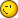The solution you get is not wrong; in fact, it is equivalent to your "by hand" solution:
C1*e^(2x) + C2*e^(-4x) + 0,5x*e^(2x) - 1/12*e^(2x) = (C1-1/12)*e^(2x)+C2*e^(-4x)+0.5xe^(2x)
Now, since C1 is an arbitrary constant, C1-1/12 is also arbitrary, so these two solutions are equivalent. ClassPad returns a slightly different solution probably due to the way it uses to solve the differential equation. I admit that the solution obtained "by hand" is more compact, but ClassPad's solution is correct as well. Btw, Mathematica's solution is identical to ClassPad's solution.

#3acid111

acid111

Casio Fan

•• Members
•• 31 posts

• Calculators:

Posted 23 April 2007 - 03:05 PM

yes, you are right !
i haven's seen it, hmm ...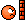ok, now i think that i can trust the solutions of the classpad...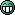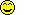thank you !

#4The_AFX_Master

The_AFX_Master

Casio Overlord

•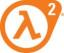•• 519 posts
• Gender:Male
• Location:Black Mesa Research Facility (sector C)
• Interests:BASIC +FORTRAN 90+ C++.....and HALF LIFE

• Calculators:
Casio Algebra FX 2.0 Plus, Casio fx 570 ms, Classpad 300, And a crowbar

Posted 23 April 2007 - 05:14 PM

I managed to solve the equation via Laplace transform on the OS 3, and i got the same result. then i guess that the calc uses laplace transforms to solve linear ODE's

#5PAP

PAP

Casio Overlord

•• Members
•• 681 posts
• Gender:Male
• Location:Somewhere in Europe.
• Interests:Computer Algebra, Numerical Analysis.

• Calculators:
ClassPad 300 (plus an old Casio model, with only a few Kb ram).

Posted 24 April 2007 - 06:49 AM

i guess that the calc uses laplace transforms to solve linear ODE's

Hmmm, not necessarily. The differential equation is linear to the dependent variable y, so it may use other methods to solve it analytically. Anyway, Laplace transforms can be used as well.

#6Guest_Logicman112_*

Guest_Logicman112_*
•• Guests

Posted 23 February 2011 - 06:05 AM

If we have a linear system with the following differential equation:
d2y/dt2+7*dy/dt+12*y=dx/dt+5*x
So H(s) = (s+5)/((s+4)*(s+3)) = -1/(s+4)+2/(s+3)--> h(t) = (-e^(-4*t)+2*e^(-3*t))*u(t)
(Please calculate the unit impulse response by Laplace transform and verify the result by yourself)
Why this answer does not satisfy the differential equation?